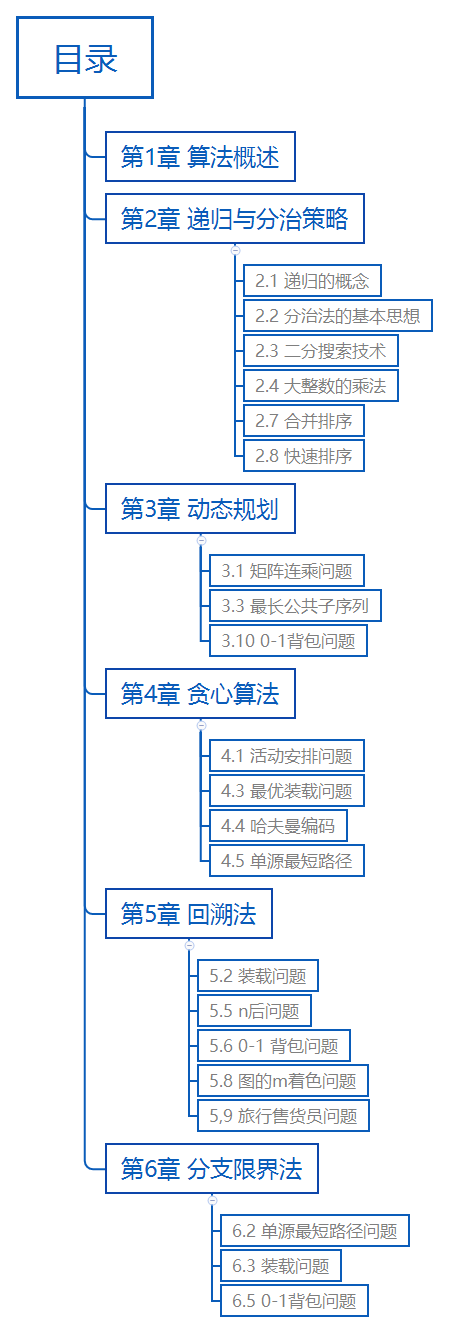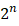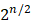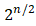# 计算机算法设计与分析（1-6章 复习笔记）1.1 算法与程序

严格地说，算法是由若干条指令组成的有穷序列，且满足下述4条性质。

1. 输入
2. 输出：至少产生一个量作为输出。
3. 确定性：每条指令清晰、无歧义。
4. 有限性：执行次数、时间有限

1.2 算法复杂性分析

1.3 NP完全性理论

分治法的思想：将一个难以直接解决的大问题分解为一些规模较小的相同问题，以便各个击破，分而治之。

2.1 递归的概念

【例2-1】阶乘函数。

n!=1                    ,n=0nn-1!   .n>0int factorial(int n){

if (n==0)

return 0;

else

return n*factorial(n-1);

}

【例2-6】Hanoi塔问题

//4个参数依次是剩余数量，起点，终点，中间点

void hanoi(int n, char a, char b, char c){

if (n==1)

move(a,b);

else

{

hanoi(n-1,a,c,b);

move(a,b);

hanoi(n-1,c,b,a);

}

}

void move(char begin,char end){

cout<<begin<<"--->"<<end<<"\n";

}

2.2 分治法的基本思想

2.3二分搜索技术

/*二分搜索 */

template<class Type>

int BinarySearch(Type a[],const Type& x, int n){

int left = 0;

int right = n-1;

while(left<=right)

{

int middle = (left+right)/2;

if (x==a[middle])

return middle;

if (x>a[middle])

left = middle+1;

if (x<a[middle])

right = middle-1;

}

return -1;

}

//递归形式

int  BinarySearch(int a[],int x,int left,int right){

if(left>right)

return -1;

int middle = (left+right)/2;

if (x==a[middle])

return middle;

if(x<a[middle])

return BinarySearch(a,x,left,middle-1);

if(x>a[middle])

return BinarySearch(a,x,middle+1,right);

}

2.4 大整数的乘法

Y分解两份：C、D

XY = （A*2n/2+B）(C*2n/2+D）

=AC*2n+((A-B)(D-C)+AC+BD)* 2n/2+BD  //利用数学变形，减少乘法次数

2.5 Strassen矩阵乘法（直接分解再乘并不能减少乘法次数，通过一些变形增加加减法次数，减少乘法次数）。

2.7 合并排序

template<class Type>

void MergeSort(Type a[],Type b[], int left, int right) {

if (left < right) {

int i = (left + right) / 2;

MergeSort(a, b,left, i);

MergeSort(a, b,i + 1, right);

Merge(a, b, left, i, right); //合并到b数组

Copy(a, b, left, right);   //复制回a数组

}

template<class Type>

void Merge(Type c[], Type d[], int l, int m, int r) {  //合并c[l:m]和c[m+1:r]到d[l:r]

int i = l, j = m + 1;

int pos = l;

while ((i <= m) && (j <= r)) {

if (c[i] <= c[j])

d[pos++] = c[i++];

else

d[pos++] = c[j++];

if (i > m) {    //第1个数组放完了，直接把第2个数组剩下的放到d

for (int q = j; q <= r; q++)

d[pos++] = c[q];

}

if (j > r) {      //第2个数组放完了，直接把第1个数组剩下的放到d

for (int q = i; q <= m; q++)

d[pos++] = c[q];

}

}

}

template<class Type>

void Copy(Type a[], Type b[], int left, int right) {

for (int i = left; i <= right; i++)

a[i] = b[i];

}

2.8 快速排序

1. 分解（Divide）：以a[p]为基准元素，a[p:r]分解为3段：a[p:q-1].a[q]]和a[q+1:r]

2.递归求解（Conquer）：使用递归对a[p:q-1]和a[q+1:r]进行排序。

3.合并（Merge）：a[p:q-1]和a[q+1:r]排序好后，不需要进行操作就已经排好序了。

template<class Type>

void QuickSort(Type a[],int p,int r){

if(p<r){

int q = Partition(a,p,r);

QuickSort(a,p,q-1);

QuickSort(a,q+1,r);

}

}

template<class Type>

int Partition(Type a[],int p, int r){

int i=p+1, j =r;

Type x = a[p];

while(true){

while(a[i]<x && i<r)   //直到找到a[i]>=x

i++;

while(a[j]>x)           //直到找到a[j]<=x

j--;

if(i>=j)

break;

swap(a[i],a[j]);    //交换a[i],a[j],使得左边都小于x，右边>x

}

swap(a[p],a[j]);

return j;

}01-17
06-011万+
01-24
06-082178
06-29
12-111487
06-102万+
01-026013
05-185285
09-167453
10-17
11-09547
07-042728
11-291119
09-10347
06-211311
08-201万+